Next: Theoretical WT predictions Up: Discreteness and its effect Previous: Equations for the free

# Statistical Quantities in Wave Turbulence

Let us consider a wavefield in a periodic square basin of side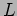and let the Fourier representation of this field be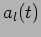where index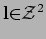marks the mode with wavenumber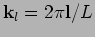on the grid in the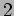-dimensional Fourier space. Discrete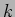-space is important for formulating the statistical problem. For simplicity let us assume that there is a cut-off wavenumber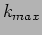so that thee is no modes with wavenumber components greater than, which is always the case in numerical simulation. In this case, the total number of modes is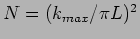and index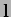will only take values in a finite box,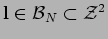which is centered at 0 and all sides of which are equal to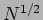. To consider homogeneous turbulence, the large box (i.e. continuous) limit,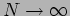, will have to be taken later.

Let us write the complex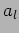as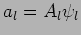where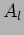is a real positive amplitude and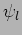is a phase factor which takes values on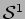, a unit circle centered at zero in the complex plane. The most general statistical object in WT  is the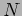-mode joint PDF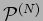defined as the probability for the wave intensities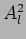to be in the range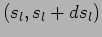and for the phase factorsto be on the unit-circle segment between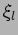and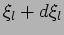for all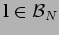.

The fundamental statistical property of the wavefield in WT is that all the amplitudesand phase factorsare independent statistical variables and that all's are uniformly distributed on. This kind of statistics was introduced in [6,4,5] and called Random Phase and Amplitude'' (RPA) field. In terms of the PDF, we say that the field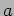is of RPA type if it can be product-factorized,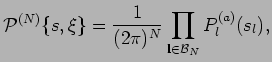(14)

where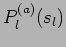is the one-mode PDF for variable.

Note that in this formulation the distributions ofremain unspecified and, therefore, the amplitudes do not have to be deterministic (as in earlier works using RPA) nor do they have to correspond to Gaussianity,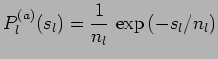(15)

where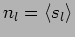is the waveaction spectrum.

Importantly, RPA formulation involves independent phase factors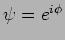and not phases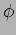. Firstly, the phases would not be convenient because the mean value of the phases is evolving with the rate equal to the nonlinear frequency correction . Thus one could not say that they are distributed uniformly from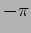to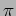''. Moreover the mean fluctuation of the phase distribution is also growing and they quickly spread beyond their initial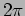-wide interval . But perhaps even more important, it was shown in  that's build mutual correlations on the nonlinear timescale whereas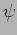's remain independent. In the present paper we are going to check this theoretical prediction numerically by directly measuring the properties of's and's.

In [6,4] RPA was assumed to hold over the nonlinear time. In  this assumption was examined a posteriori, i.e. based on the evolution equation for the multi-point PDF. Note that only the phase randomness is necessary for deriving this equation, whereas both the phase and the amplitude randomness are required for the WT closure for the one-point PDF or the kinetic equation for the spectrum. This fact allows to prove that, if valid initially, the RPA properties survive in the leading order in small nonlinearity and in the large-box limit . Such an approximate leading-order RPA is sufficient for the WT closure.Next: Theoretical WT predictions Up: Discreteness and its effect Previous: Equations for the free
Dr Yuri V Lvov 2007-01-16